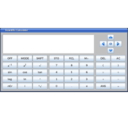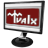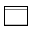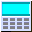# jscicalc2 - Java Scientific Calculator - A java-based scientific calculator

1.1.1

This is a simple scientific calculator programmed in Java. You can use it to calculate trigonometric and inverse-trigonometric values, permutations & combinations, logarithms & exponents, and many other functions. It is also capable of working with complex numbers, and Binary, Hexadecimal and Octal numbers.

Open source
OS:
Windows 7/8.1/8/XP/10
Date:
File size:
362.19 KB
Category rank:
593
All software rank:
26 270

## More about jscicalc2 - Java Scientific Calculator - A java-based scientific calculator

You can download the installation package 362.19 KB of jscicalc2 - Java Scientific Calculator - A java-based scientific calculator v1.1.1 from this website. This program is created by John D Lamb and available free of charge. Users can find it in Education category, namely Science. The version 1.1 is officialy supported on Windows 7 operating systems. The antivirus check of the direct download link proved it to be safe.Download
jscicalc2 - Java Scientific Calculator - A java-based scientific calculator 1.1.1

## Comments on jscicalc2 - Java Scientific Calculator - A java-based scientific calculator

Thank you for rating the program!

### Useful softwareScientific Calculator Precision 54
It allows you to perform complex mathematical calculations.DreamCalc Scientific Calculator
DreamCalc Scientific Calculator (Scientific Edition).DreamCalc Scientific Graphing Calculator
DreamCalc is an Advanced Scientific and Graphing Calculator for Windows.eCalc Scientific
Scientific calculator with all the math functions - from simple to complex.HEXelon MAX
One of the best free scientific calculators and converters.